Open in App
Not now

# Dynamically Rename Multiple Columns in PySpark DataFrame

• Last Updated : 07 Mar, 2023

In this article, we are going to learn how to dynamically rename multiple columns in Pyspark data frame in Python.

A data frame that is equivalent to a relational table in Spark SQL, and can be created using various functions in SparkSession is known as Pyspark data frame. While working in Pyspark, we notice numerous times the naming of columns is not in the way we want for applying functions on the columns. These columns can be renamed dynamically through various ways in Pyspark, such as through loops, through reduce() function, etc. In this article, we have discussed all the ways to dynamically rename multiple columns in PySpark data frame.

### What is meant by Dynamically renaming multiple columns in PySpark data frame?

The process of changing the names of multiple columns of Pyspark data frame during run time is known as dynamically renaming multiple columns in Pyspark data frame. The renaming is done in order to call the columns by their names rather than index and apply appropriate functions on the columns.

## Methods to dynamically rename multiple columns in PySpark data frame:

• Using loops
• Using reduce() function
• Using the alias() function
• Using quinn() function
• Using toDF() function

### Method 1: Using loops

A process that can be used to repeat a certain part of code is known as looping. In this method, we will see how we can dynamically rename multiple columns in PySpark data frame created by the user or read through the CSV file. What we will do is create a loop to rename the multiple columns one by one.

Stepwise implementation of dynamically rename multiple columns using loops:

Step 1: First of all, import the required library, i.e., SparkSession. The SparkSession library is used to create the session.

`from pyspark.sql import SparkSession`

Step 2: Now, create a spark session using the getOrCreate() function.

`spark_session = SparkSession.builder.getOrCreate()`

Step 3: Then, read the CSV file or create the data frame using the createDataFrame() function for which you want to dynamically rename the multiple column names.

```data_frame=csv_file = spark_session.read.csv('#Path of CSV file',
sep = ',', inferSchema = True, header = True)```

or

```data_frame=spark_session.createDataFrame([(column_1_data), (column_2_data), (column_3_data)],
['column_name_1', 'column_name_2', 'column_name_3'])```

Step 4: Later on, obtain all the columns in the list using the columns function.

`total_columns=data_frame.columns`

Step 5: Further, run a loop to dynamically rename multiple columns in Pyspark data frame using prefix, suffix or doing any other changes.

```for i in range(len(total_columns)):
data_frame=data_frame.withColumnRenamed(total_columns[i], 'class_'+ total_columns[i])
#or
data_frame=data_frame.withColumnRenamed(total_columns[i], total_columns[i].replace('_','__'))```

Step 6: Finally, display the updated data frame.

`data_frame.show()`

Example:

In this example, we have read the CSV file (link), i.e., basically a data set of 5*5 as follows: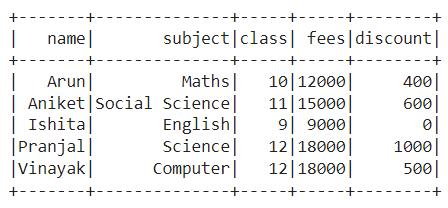Then, we got all the column names in the list. Further, we have run a loop to rename multiple column names with the prefix ‘class_‘. Also, we have run a loop to rename multiple column names to replace ‘_’ in the names of the columns with ‘__’ and displayed the data frame.

## Python3

 `# Python program to dynamically rename multiple` `# columns in PySpark DataFrame using loops`   `# Import the libraries SparkSession library` `from` `pyspark.sql ``import` `SparkSession`   `# Create a spark session using getOrCreate() function` `spark_session ``=` `SparkSession.builder.getOrCreate()`   `# Read the CSV file` `data_frame ``=` `csv_file ``=` `spark_session.read.csv(` `    ``'/content/class_data.csv'``,` `    ``sep``=``','``, inferSchema``=``True``, header``=``True``)`   `# Get all the columns of data frame in a list` `total_columns ``=` `data_frame.columns`   `# Run loop to dynamically rename multiple columns` `# in PySpark DataFrame with prefix 'class_'` `for` `i ``in` `range``(``len``(total_columns)):` `    ``data_frame ``=` `data_frame.withColumnRenamed(` `        ``total_columns[i],` `        ``'class_'` `+` `total_columns[i])`   `# Get all the columns of data frame in a list` `total_columns1 ``=` `data_frame.columns`   `# Run loop to dynamically rename multiple columns` `# in PySpark DataFrame by replacing '_' with '__'` `for` `j ``in` `range``(``len``(total_columns1)):` `    ``data_frame ``=` `data_frame.withColumnRenamed(` `        ``total_columns1[j],` `        ``total_columns1[j].replace(``'_'``, ``'__'``))`   `# Display the data frame` `data_frame.show()`

Output: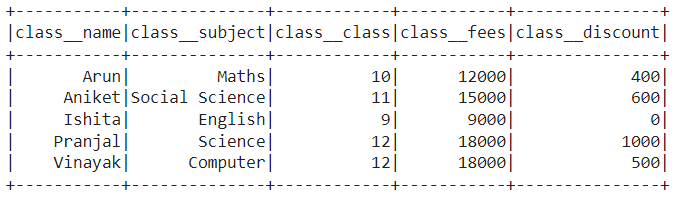### Method 2: Using reduce() function

An aggregate action function that is used to calculate the min, the max, and the total of elements in a dataset is known as reduce() function. In this method, we will see how we can dynamically rename multiple columns in Pyspark data frame using reduce() function created by the user or read through the CSV file. What we will do is apply the reduce() function on the data frame with the function to rename the multiple columns using the withColumnRenamed() function.

Stepwise implementation of dynamically rename multiple columns using reduce() function:

Step 1: First of all, import the required libraries, i.e., SparkSession and functools. The SparkSession library is used to create the session while the functools is a function that acts on or returns other functions.

```from pyspark.sql import SparkSession
import functools```

Step 2: Now, create a spark session using the getOrCreate() function.

`spark_session = SparkSession.builder.getOrCreate()`

Step 3: Then, read the CSV file or create the data frame using the createDataFrame() function for which you want to dynamically rename the multiple column names.

```data_frame=csv_file = spark_session.read.csv('#Path of CSV file',
sep = ',', inferSchema = True, header = True)```

or

```data_frame=spark_session.createDataFrame([(column_1_data), (column_2_data), (column_3_data)],
['column_name_1', 'column_name_2', 'column_name_3'])```

Step 4: Further, dynamically rename multiple columns in PySpark data frame using prefix, suffix or doing any other changes using reduce() function.

```data_frame = functools.reduce(lambda data_frame,
idx: data_frame.withColumnRenamed(list(data_frame.schema.names)[idx],
list(data_frame.schema.names)[idx] + '_suffix'),
range(len(list(data_frame.schema.names))), data_frame)```

Step 5: Later on, dynamically rename multiple columns in PySpark data frame by replacing some characters using replace and reduce() function.

```data_frame = functools.reduce(lambda data_frame,
idx: data_frame.withColumnRenamed(list(data_frame.schema.names)[idx],
list(data_frame.schema.names)[idx].replace('#character','#other-character')),
range(len(list(data_frame.schema.names))), data_frame)```

Step 6: Finally, display the updated data frame.

`data_frame.show()`

Example:

In this example, we have read the CSV file (link),  i.e., basically a data set of 5*5 as follows:Then, we have dynamically renamed multiple column names with the suffix ‘_suffix‘ using the reduce() function. Also, we have replaced ‘_’ in the names of the columns with ‘__’ using the reduce() function and displayed the data frame.

## Python3

 `# Python program to dynamically rename multiple ` `# columns in PySpark DataFrame using reduce function`   `# Import the libraries SparkSession and functools libraries` `from` `pyspark.sql ``import` `SparkSession` `import` `functools`   `# Create a spark session using getOrCreate() function` `spark_session ``=` `SparkSession.builder.getOrCreate()`   `# Read the CSV file` `data_frame``=``csv_file ``=` `spark_session.read.csv(` `  ``'/content/class_data.csv'``,` `  ``sep ``=` `','``, inferSchema ``=` `True``, header ``=` `True``)`   `# Dynamically rename multiple columns in PySpark DataFrame` `# with suffix '_suffix' using reduce function` `data_frame ``=` `functools.``reduce``(``lambda` `data_frame,` `             ``idx: data_frame.withColumnRenamed(` `               ``list``(data_frame.schema.names)[idx],` `               ``list``(data_frame.schema.names)[idx] ``+` `'_suffix'``),` `               ``range``(``len``(``list``(data_frame.schema.names))),` `                              ``data_frame)`   `# Dynamically rename multiple columns in PySpark DataFrame by` `# replacing '_' with '__' using reduce function` `data_frame ``=` `functools.``reduce``(``lambda` `data_frame,` `             ``idx: data_frame.withColumnRenamed(` `               ``list``(data_frame.schema.names)[idx],` `               ``list``(data_frame.schema.names)[idx].replace(``'_'``,``'__'``)),` `               ``range``(``len``(``list``(data_frame.schema.names))),` `                              ``data_frame)`   `# Display the data frame` `data_frame.show()`

Output: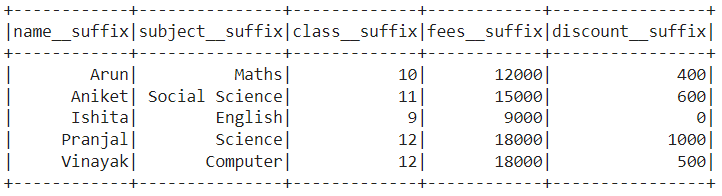### Method 3: Using the alias() function

A method that is used to make a special significance for a column or table in Pyspark that is more often readable and shorter is known as the alias() function. In this method, we will see how we can dynamically rename multiple columns in PySpark data frame created by the user or read through the CSV file. What we will do is take the name of all the columns in the list and rename them by adding suffix or prefix to all the values of that list or doing any other changes, with further updating the data frame with new column names.

#### Stepwise implementation of dynamically rename multiple columns using alias:

Step 1: First of all, import the required libraries, i.e., SparkSession and col. The SparkSession library is used to create the session while the col is used to return a column based on the given column name.

```from pyspark.sql import SparkSession
from pyspark.sql.functions import col```

Step 2: Now, create a spark session using the getOrCreate() function.

`spark_session = SparkSession.builder.getOrCreate()`

Step 3: Then, read the CSV file or create the data frame using the createDataFrame() function for which you want to dynamically rename the multiple column names.

```data_frame=csv_file = spark_session.read.csv('#Path of CSV file',
sep = ',', inferSchema = True, header = True)```

or

```data_frame=spark_session.createDataFrame([(column_1_data), (column_2_data), (column_3_data)],
['column_name_1', 'column_name_2', 'column_name_3'])```

Step 4: Further, dynamically rename multiple columns in PySpark data frame using prefix, suffix or doing any other changes using alias.

`updated_columns = [col(col_name).alias("prefix_" + col_name + "_suffix")  for col_name in data_frame.columns]`

Step 5: Later on, update the data frame according to the new column names.

`data_frame1=data_frame.select(*updated_columns)`

Step 6: In this step, we will replace some characters of the names of the columns with some other characters using replace() function.

`updated_columns1 = {c:c.replace('_','__') for c in data_frame1.columns if '_' in c}`

Step 7: Finally, update the column with the new column names and display the data frame.

`data_frame1.select([col(c).alias(updated_columns1.get(c, c)) for c in data_frame1.columns]).show()`

Example:

In this example, we have read the CSV file (link), i.e., basically a data set of 5*5 as follows:Then, we have dynamically renamed multiple column names with the prefix ‘prefix_‘ and the suffix ‘_suffix‘ using the alias. Also, we have replaced ‘_‘ in the names of the columns with ‘__‘ using the replace() function. Finally, we have set the renamed column names to the data frame and displayed the data frame.

## Python3

 `# Python program to dynamically rename multiple ` `# columns in PySpark DataFrame using alias`   `# Import the libraries SparkSession and col libraries` `from` `pyspark.sql ``import` `SparkSession` `from` `pyspark.sql.functions ``import` `col`   `# Create a spark session using getOrCreate() function` `spark_session ``=` `SparkSession.builder.getOrCreate()`   `# Read the CSV file` `data_frame``=``csv_file ``=` `spark_session.read.csv(` `  ``'/content/class_data.csv'``,` `  ``sep ``=` `','``, inferSchema ``=` `True``, header ``=` `True``)`   `# Dynamically rename multiple columns in PySpark ` `# DataFrame with prefix 'prefix_' and suffix '_suffix' using alias` `updated_columns ``=` `[col(col_name).alias(` `  ``"prefix_"` `+` `col_name ``+` `"_suffix"``)` `            ``for` `col_name ``in` `data_frame.columns]`   `# Creating a new data frame by replacing ` `# column names with prefix or suffix or both` `data_frame1``=``data_frame.select(``*``updated_columns)`   `# Replace '_' with '__' in the naming of the columns` `updated_columns1 ``=` `{c:c.replace(``'_'``,``'__'``)` `                    ``for` `c ``in` `data_frame1.columns ``if` `'_'` `in` `c}`   `# Display the updated data frame with new column names using alias` `data_frame1.select([col(c).alias(updated_columns1.get(c, c))` `                    ``for` `c ``in` `data_frame1.columns]).show()`

Output: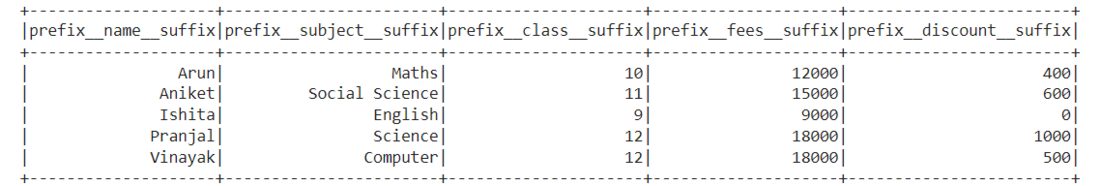### Methods 4: Using quinn() function

The way to validate data frames, extends core classes, defines data frame transformations, and provides SQL functions is known as quinn() function. In this method, we will see how we can dynamically rename multiple columns in PySpark data frame using quinn() function created by the user or read through the CSV file. What we will do is create a new function and call that function using quinn to rename the multiple columns using the with_columns_renamed() function.

#### Stepwise implementation of dynamically rename multiple columns using quinn:

Step 1: First of all, import the required libraries, i.e., SparkSession and quinn. The SparkSession library is used to create the session while the quinn is used to validate data frames, extends core classes, defines data frame transformations, and provides SQL functions.

```from pyspark.sql import SparkSession
import quinn```

Step 2: Now, create a spark session using the getOrCreate() function.

`spark_session = SparkSession.builder.getOrCreate()`

Step 3: Then, read the CSV file or create the data frame using the createDataFrame() function for which you want to dynamically rename the multiple column names.

```data_frame=csv_file = spark_session.read.csv('#Path of CSV file',
sep = ',', inferSchema = True, header = True)```

or

```data_frame=spark_session.createDataFrame([(column_1_data), (column_2_data), (column_3_data)],
['column_name_1', 'column_name_2', 'column_name_3'])```

Step 4: Further, dynamically rename multiple columns in PySpark data frame using prefix, suffix, replacing characters or doing any other changes by creating a function.

```def function_quinn(s):
s=s+'_suffix'
return s.replace('_', '__')```

Step 5: Moreover, call the function to set the renamed columns to data frame using quinn.

`updated_df = data_frame.transform(quinn.with_columns_renamed(function_quinn))`

Step 6: Finally, display the updated data frame setting the renamed column names.

`updated_df.show()`

Example:

In this example, we have read the CSV file (link),  i.e., basically a data set of 5*5 as follows:Then, we have dynamically renamed multiple column names with the prefix ‘_suffix‘ and then replacing ‘_‘ with ‘__‘ by creating a function. Finally, we have called the function to set the renamed column names to the data frame using quinn and displayed the data frame.

## Python3

 `# Python program to dynamically rename multiple ` `# columns in PySpark DataFrame using quinn`   `# Import the SparkSession and quinn libraries` `from` `pyspark.sql ``import` `SparkSession` `import` `quinn`   `# Create a spark session using getOrCreate() function` `spark_session ``=` `SparkSession.builder.getOrCreate()`   `# Read the CSV file` `data_frame``=``csv_file ``=` `spark_session.read.csv(` `  ``'/content/class_data.csv'``,` `  ``sep ``=` `','``, inferSchema ``=` `True``, header ``=` `True``)`   `# Create a function to add suffix and then replace` `# characters in column name` `def` `function_quinn(s):` `    ``s``=``s``+``'_suffix'` `    ``return` `s.replace(``'_'``, ``'__'``)`   `# Dynamically rename multiple columns in PySpark` `# DataFrame by calling the function` `updated_df ``=` `data_frame.transform(` `        ``quinn.with_columns_renamed(function_quinn))`   `# Display the updated data frame` `updated_df.show()`

Output: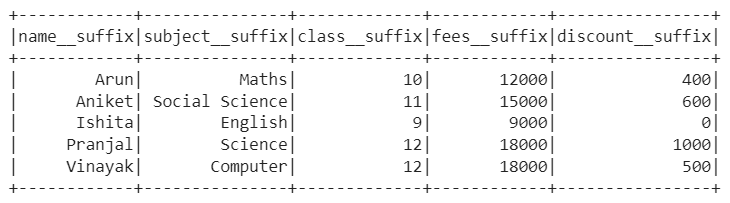### Methods 5: Using the toDF function

A method in PySpark that is used to create a Data frame in PySpark is known as the toDF() function. In this method, we will see how we can dynamically rename multiple columns using the toDF() function on all the columns of the data frame created by the user or read through the CSV file. What we will do is create a new data frame and put the values of an existing data frame in the new data frame with the new column names.

#### Stepwise implementation to dynamically rename multiple columns using the toDF() function:

Step 1: First of all, import the required libraries, i.e., SparkSession. The SparkSession library is used to create the session.

`from pyspark.sql import SparkSession`

Step 2: Now, create a spark session using the getOrCreate() function.

`spark_session = SparkSession.builder.getOrCreate()`

Step 3: Then, either create the data frame or read the CSV file for which you want to rename the column names with prefixes or suffixes.

```data_frame=spark_session.createDataFrame([(column_1_data), (column_2_data), (column_3_data)],
['column_name_1', 'column_name_2', 'column_name_3'])```

or

```data_frame=csv_file = spark_session.read.csv('#Path of CSV file',
sep = ',', inferSchema = True, header = True)```

Step 4: Further, define the new column names which you want to give to all the columns.

`columns=['new_column_name_1','new_column_name_2','new_column_name_3']`

Step 5: Finally, use the function toDF() and assign the names to the data frame and display it.

`data_frame.toDF(*columns).show()`

Example:

In this example, we have read the CSV file (link),  i.e., basically a data set of 5*5 as follows:Then, we defined a list with new column names and allocated those names to the columns of the data frame using the toDF() function.

## Python3

 `# Python program to dynamically rename multiple ` `# columns in PySpark DataFrame using toDF function`   `# Import the libraries SparkSession library` `from` `pyspark.sql ``import` `SparkSession`   `# Create a spark session using getOrCreate() function` `spark_session ``=` `SparkSession.builder.getOrCreate()`   `# Read the CSV file` `data_frame``=``csv_file ``=` `spark_session.read.csv(` `  ``'/content/class_data.csv'``,` `   ``sep ``=` `','``, inferSchema ``=` `True``, header ``=` `True``)`   `# Define the new column names to be given to data frame` `columns ``=` `[``'Class_Name'``, ``'Class_Subject'``,` `           ``'Class_Class'``, ``'Class_Fees'``, ``'Class_Discount'``]`   `# Allocate the new column names to the data frame and display it` `data_frame.toDF(``*``columns).show()`

Output:My Personal Notes arrow_drop_up
Related Articles